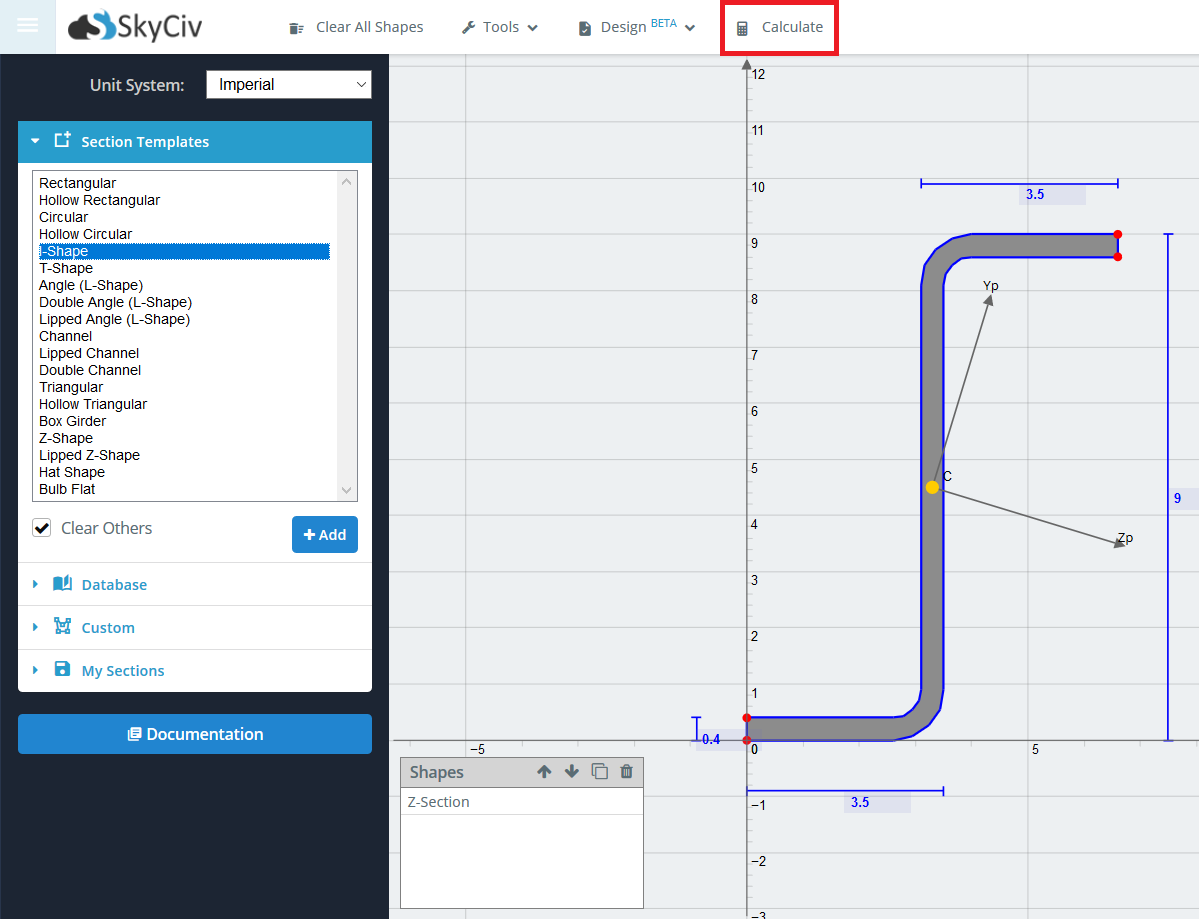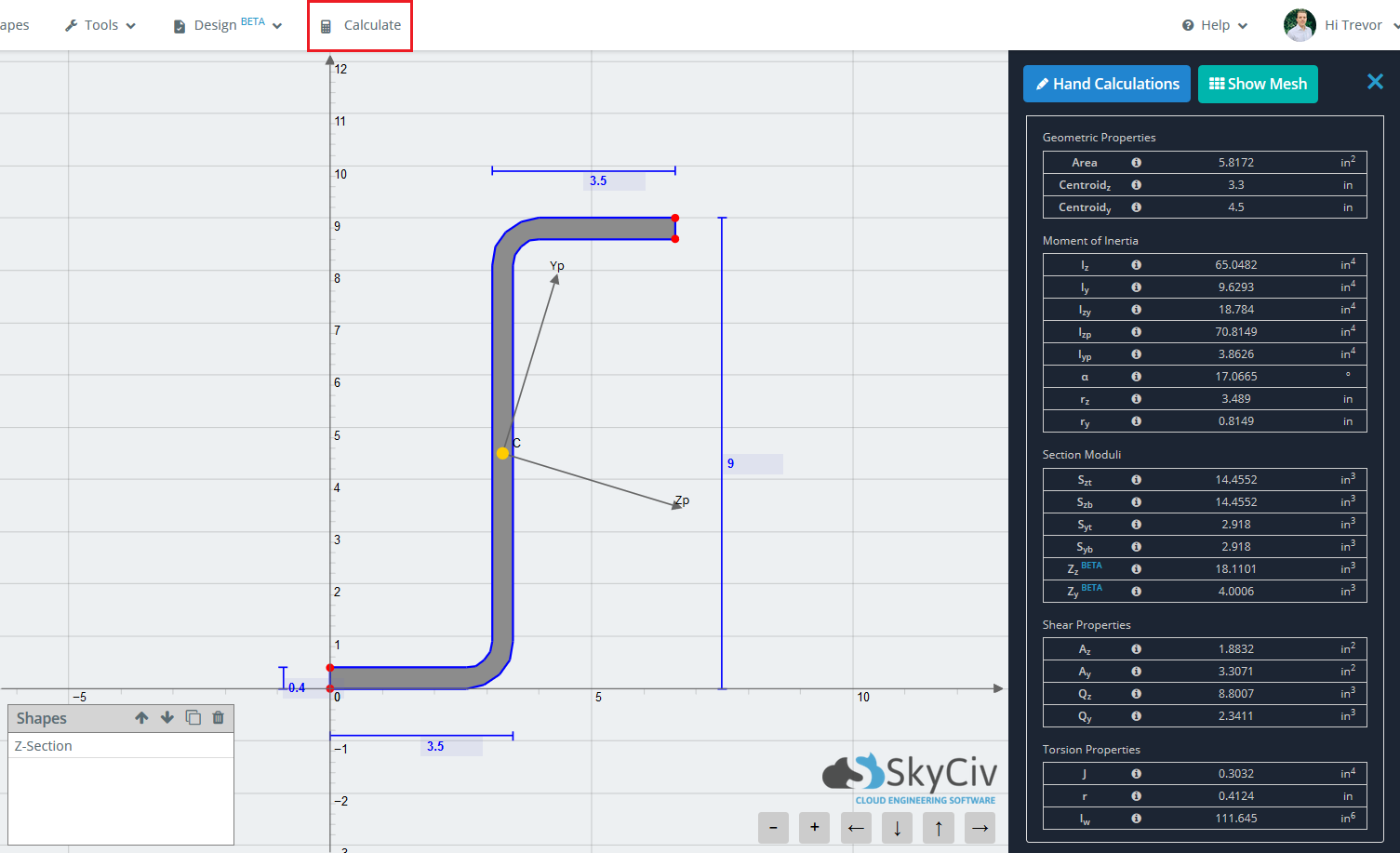SkyCiv文档

1. SkyCiv版块生成器
2. 结果
3. 标准结果

# 标准结果

The main purpose of the Section Builder is to calculate the various section properties of your section. The standard results refer to the results of a section when ignoring material property differences.

When you’ve finished building your section, simply click ‘Calculateon the top toolbar to determine the section properties. Note that the short wait time associated with each calculate is due to the Finite Element Analysis and Meshing that occurs in the background in order to solve for torsion constant, torsion radius, and warping constant. By using a FEA method to calculate these properties, a higher accuracy is achieved. Less robust programs only use approximate equations on the standard sections for these properties and fail to solve when a non standard section (such as the cross beam below) is entered.The results are generally broken down into 5 main categories.

• 几何特性
• 质心 (与)
• 质心 (和)
• Moment of Inertia Properties
• 从 – 绕 Z 轴的转动惯量
• y – 绕 Y 轴的转动惯量
• Izy – 惯性矩
• IzpMoment of Inertia about the principal Z-axis
• IypMoment of Inertia about the principal Y-axis
• 一种 – angle of rotation of principal axis
• z – Radius of Gyration about the Z-axis
• 瑞 – Radius of Gyration about the Y-axis
• 截面模数
• 件 – Elastic Section Modulus about the Z-axis to the top of the section
• 斯巴达 – Elastic Section Modulus about the Z-axis to the bottom of the section
• 眼睛 – Elastic Section Modulus about the Y-axis to the top of the section
• 西伯 – Elastic Section Modulus about the Y-axis to the bottom of the section
• ZzPlastic Section Modulus about the Z-axis
• ZyPlastic Section Modulus about the Y-axis
• 剪切特性
• AzShear Area about Z axis
• AyShear Area about Y axis
• Qz – Static Moment of Area about the Z neutral axis
• Qy – Static Moment of Area about the Y neutral axis
• Torsion Properties
• Ĵ – 扭转常数
• [R – 扭转半径
• 和 – 翘曲常数